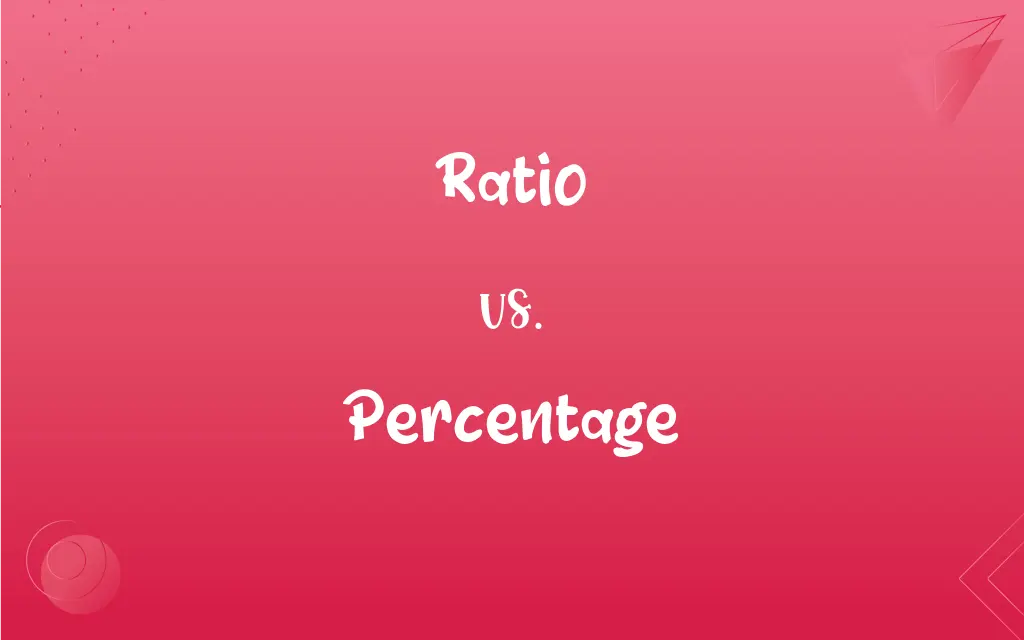# Ratio vs. Percentage## Ratio and Percentage Definitions

#### Ratio

Relation in degree or number between two similar things.

#### Percentage

A fraction or ratio with 100 understood as the denominator; for example, 0.98 equals a percentage of 98.

#### Ratio

The relative value of silver and gold in a currency system that is bimetallic.

#### Percentage

The result obtained by multiplying a quantity by a percent.

#### Ratio

(Mathematics) A relationship between two quantities, normally expressed as the quotient of one divided by the other; for example, the ratio of 7 to 4 can be written 7:4 or 7/4. A ratio can often also be expressed as a decimal or percentage.

#### Percentage

A proportion or share in relation to a whole; a part
The hecklers constituted only a small percentage of the audience.

#### Ratio

A number representing a comparison between two named things.

#### Percentage

An amount, such as an allowance, duty, or commission, that varies in proportion to a larger sum, such as total sales
Work for a percentage.

#### Ratio

(arithmetic) The relative magnitudes of two quantities (usually expressed as a quotient).

#### Percentage

There is no percentage in work without pay.

#### Ratio

(legal) ratio decidendi

#### Percentage

The amount, number or rate of something, regarded as part of a total of 100; a part of a whole.
A high percentage of secondary school leavers take a gap year.

#### Ratio

(Internet) The number of comments to a post or other expression on social media relative to the number of likes; a high ratio suggests disagreement with the contents of the original post.

#### Percentage

A share of the sales, profits, gross margin or similar.
She gets a percentage for every vacuum cleaner sold.

#### Ratio

To respond to a post or message on social media in a greater number than the number of likes the post receives, especially to condemn or mock the original poster.
The politician's post was quickly ratioed due to its controversial nature.

#### Percentage

There was no percentage in staying at home.

#### Ratio

Indicates disagreement with a post the user disagrees with or dislikes.
L + ratio

#### Percentage

A certain rate per cent; the allowance, duty, rate of interest, discount, or commission, on a hundred.

#### Ratio

The relation which one quantity or magnitude has to another of the same kind. It is expressed by the quotient of the division of the first by the second; thus, the ratio of 3 to 6 is expressed by

#### Percentage

A proportion multiplied by 100

#### Ratio

Hence, fixed relation of number, quantity, or degree; rate; proportion; as, the ratio of representation in Congress.

#### Percentage

Assets belonging to or due to or contributed by an individual person or group;
He wanted his share in cash

#### Ratio

The relative magnitudes of two quantities (usually expressed as a quotient)